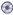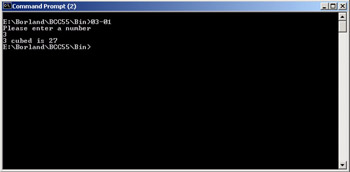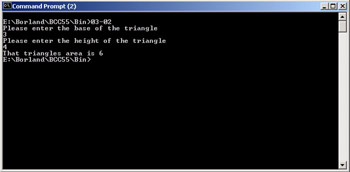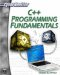# Creating and Calling Functions

Obviously you could simply put everything into the main function and it would, indeed, compile and run, but this would quickly become unwieldy. In fact, your program would certainly become completely unmanageable and unreadable by the time it even reached a few dozen lines of code. Fortunately you don’t have to do that. You can create separate functions to handle these other items. There are a few idiosyncrasies regarding function creation in C++. The following list summarizes the requirements.

1. The function must have a return type. It may be void if the function returns nothing.

2. The function must have a valid name.

3. The function must have parentheses for a list of parameters past to it, even if the parentheses are empty.

4. The function must either be written before it’s called, or it must be prototyped.

The first three you already know about, but the fourth rule may sound quite confusing. What does it mean to prototype a function? Essentially the problem is that the C++ compiler cannot call a function it does not know about. You have to let the compiler know what that function is and what it does before you can call it. One way to do this is to make sure you write out the function in your file, before you call it. This quickly becomes quite cumbersome. Therefore, C++ provides another way to do this. That method is prototyping. All you do to prototype a function is to write its declaration line at the beginning of the source file. This would probably be much clearer with an example.

### Example 4.1

In this example we will create a function that simply cubes any number passed to it and returns the answer. That function is prototyped and then called from the main function.

Step 1: Open your favorite text editor and type in the following code. You will then save it to a file called04-01.cpp.

`// Include statements #include <iostream>  using namespace std; // function prototypes. float cube_number(float num); int main() {  float number;  float number4;  cout << "Please enter a number \n";  cin >> number;    number4 = cube_number(number);  cout << number << " cubed is " << number4;  return 0;  } float cube_number(float num) {  // this function simply takes a number, cubes it,  // then returns the answer  float answer; return answer; }`

Step 2: Compile the code. (See Appendix D if you need more instruction on how to compile your programs.)

Step 3: You can now run your program by typing in 04-01 at the command prompt. If all goes well (no compile errors), then you will see something like what is shown in Figure 4.1.Figure 4.1: Basic functions.

This code contains the essentials of writing, prototyping, and using functions. Let’s examine it piece by piece to make sure you understand what is happening. The first line is simply an include statement. We include the iostream header file, so that you can do input and output (as you first saw in Chapter 2). The second line identifies that we wish to use the standard namespace, thus giving us access to cout, cin, endl, and any other function that may reside in iostream. Next is our prototype for our function. It is literally an exact copy of that functions declaration line. It lets the compiler know that there is a function later in the code. It’s defined so that it takes certain parameters and returns a certain value. (If you see a call to this function, before you get to the part where the function is written, don’t panic!).

 Hint! The main function does not need to be prototyped; its declaration line is built into C++. Also, functions that are included in one of the C++ header files do not need to be prototyped (such as cin and strcpy from Chapter 2).

Once you get into the main function of this program, you see a few variables declared. One variable, number, stores whatever number the user types in. The second variable, number4, is designed to store the answer that the cube_number function returns. When you call cube_number, because it returns a value, you must set the function on the righthand side of an equation with an appropriate variable. The following is a generic example. Some variable, of the same data type as the function’s return type, is set equal to the function name. For example, float:

`f = myfunc()`

This example uses a variable of type double (the same type as the function is declared to return) and sets that variable equal to that function. This expression will cause the function to be called and its value stored in the variable in question.

You should also pay particular attention to the function cube_number. Notice that it does have a parameter. Beginning programmers sometimes have trouble understanding what to pass as a parameter. The simple rule of thumb is this: If you were going to ask some person to perform the same task you are asking the function to perform, what would you have to give them? The answer to that is the parameters you will need to pass to the function in question. If you were asked to cube a number, someone would have to give you the number to cube. Thus, if you ask a function to cube a number, you have to pass it that number. Parameters are simply values stored in variables that you pass to functions. They are the raw “stuff” that functions use to produce the product desired. Parameters are also frequently called “arguments.” The words argument and parameter are used interchangeably.

Let’s take a look at another example.

### Example 4.2

In this example, the function takes two parameters to compute the area of a triangle. The formula for this is area = 1/2 of the base of the triangle, multiplied by the height of the triangle.

`   A = 1/2 (b * h)`

Step 1: Enter the following code in your favorite text editor. Save it in a file called04-02.cpp.

`#include <iostream>  using namespace std; // function prototypes float triangle_area(float b, float h); int main() {  float base, height, area;  // get the user input  cout << "Please enter the base of the triangle  \n";  cin>> base;  cout << "Please enter the height of the    triangle\n";  cin >> height;  area = triangle_area(base , height);  cout << "That triangles area is " << area;  return 0; } float triangle_area(float b, float h) {   float a;   a = .5 * (b * h);   return a; } `

Step 2: Compile the code.

Step 3: Run the code. You should see something similar to what is presented in Figure 4.2.Figure 4.2: Basic functions 2.

This example is a lot like the first example, with the exception that the function triangle_area takes two parameters. A function may take zero parameters, one parameter, two parameters, and so on. Theoretically you can have a long list of parameters (also called arguments), dozens if you wish. However, as a rule of thumb, if you need more than three or four parameters, you may wish to rethink your code.

You should note that with the exception of the two parameters instead of one, this program is very much like the first one. It begins with include statements, prototypes any functions other than main, has the main function, and then has other functions. You don’t have to declare the main function first. However, it seems logical to put that function first, because that is where the entire program is going to start. Also remember that you can have a function call another function. In fact, in both of our examples, the main function called the second function.C++ Programming Fundamentals (Cyberrookies)
ISBN: 1584502371
EAN: 2147483647
Year: 2005
Pages: 197
Authors: Chuck Easttom